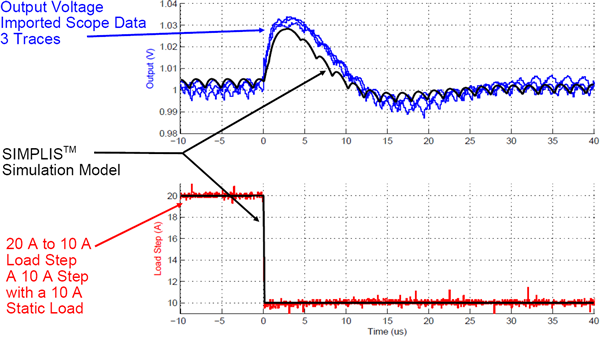# Accuracy of SIMPLIS PWL Models

When used to model switching power systems, Piecewise Linear (PWL) models provide a high degree of accuracy. From a numeric perspective, SIMPLIS finds an accurate solution when solving the simulation. This accuracy includes the effect of ripple voltages in the control path, which is something an averaged model overlooks. As a result, the SIMPLIS AC analysis can closely match experimental data when the model has been carefully constructed to include the minute details of the converter operation.

The three examples described below compare the SIMPLIS results with actual hardware testing.

## AC Example: Self Oscillating Flyback Converter

The following graphs compare the SIMPLIS AC analysis in red with bench measurements on a hardware prototype. These results were taken from the circuit example in the What is SIMPLIS? topic. The files can be downloaded here: SelfOscillatingConverter_POP_AC_Tran.zip.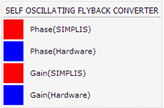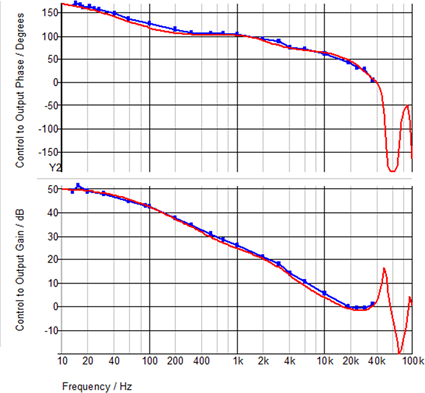## Transient Example: Quasi-Resonant Flyback Converter

This example is from a Quasi-Resonant Flyback Converter used in an AC adapter application. The main MOSFET and output rectifiers in the example were automatically converted from the SPICE models with the Model Parameter Extraction algorithms. The resulting SIMPLIS model closely matches the hardware measurements.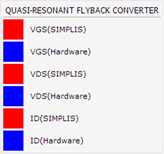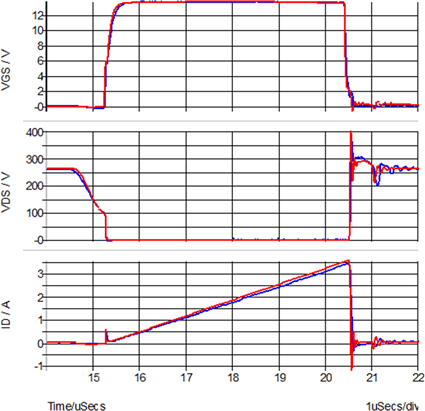## Transient Example: Single Phase SyncBuck

This example comes from a single-phase, digitally-controlled, synchronous Buck converter. These oscilloscope images were taken with three triggers of the hardware measurement, and the SIMPLIS simulation points were overlaid on the hardware data.

### Step load increase from 10A to 20A

Time-domain simulation vs. experimental results

Digitally Controlled Synchronous Buck Converter - Step Load Increase

### Step load decrease from 20A to 10A

Time-domain simulation vs. experimental results